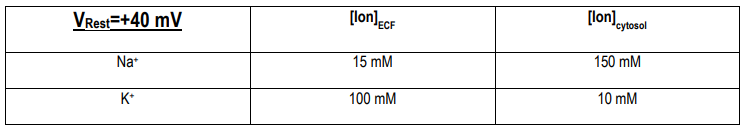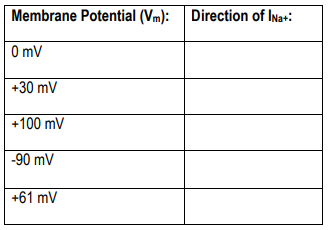Clutch Prep is now a part of Pearson
Ch. 5 - Membrane DynamicsWorksheetSee all chapters

# The Resting Membrane Potential III: The Nernst and Goldman-Hodgkin-Katz Equations

See all sections

Concept #1: The Nernst Equation

Concept #2: Using the Goldman-Hodgkin-Katz Equation

Practice: Assume that, for a given cell: [Ca2+]extracellular fluid=120 mM and [Ca2+]cytosol=1.2 mM. Which of the following is true for this cell?

Practice: A typical neuron is sitting at Vrest=- 70 mV. Many Na+ channels in the neuron’s membrane open and stay open. [Na+]outside=150 mM and [Na+]inside=15 mM. After a new equilibrium is reached, you measure the new membrane potential. Which of the following is likely to be the value of that new membrane potential?

Practice: You discover an alien neuron and take some measurements. At rest, the membrane is most permeable to which ion?Practice: A cell is initially at Vm=0 mV. The concentrations of K+ inside and outside the cell are: [K+]out=5 mM, [K+]in=150 mM. If a channel for K+ opens, will K+ flow into or out of the cell?

Practice: Complete the table for a cell with [Na+]out=150 mM and [Na+]in=15 mM.